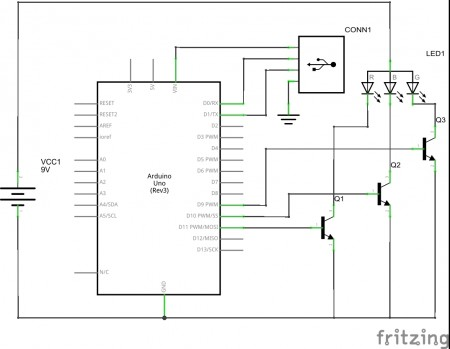# Ambient Light

So, I just wanted an Ambient Light for my TV.
Could be quite easy.
I got an Arduino and a Cheap RGB LED Strip, here is the working diagram:Arduino gets Info from USB, and switches the right RGB Vvalues accordingly.

Now my laptop has just to execute this script:
``` import java.awt.Robot; import java.awt.AWTException; import java.awt.event.InputEvent; import java.awt.image.BufferedImage; import java.awt.Rectangle; import java.awt.Dimension; import processing.serial.*; Serial port; Robot robby; void setup() { port = new Serial(this, Serial.list(),9600); size(100, 100); try { robby = new Robot(); } catch (AWTException e) { println("Robot class not supported by your system!"); exit();}}```

``` void draw() {int pixel; float r=0; float g=0; float b=0; BufferedImage screenshot = robby.createScreenCapture(new Rectangle(new Dimension(1920,1080))); int i; int j; for(i=0;i<1920; i=i+2){ for(j=0; j<136;j=j+2){ pixel = screenshot.getRGB(i,j); r = r+(int)(255&(pixel>>16)); g = g+(int)(255&(pixel>>8)); b = b+(int)(255&(pixel)); }} ```

```r=r/(960*68); g=g/(960*68); b=b/(960*68); port.write(0xff); port.write((byte)(r)); port.write((byte)(g)); port.write((byte)(b)); delay(10); background(r,g,b);} ```

It works so perfect!
The light is bright, the colors change nice and fast.

Only problem..my laptop is way to slow!
This transforms every movie into a dia show..
And I even just get the RGB info of the upper 12% of the screen.

Need to
– find new software
– get new laptop
– find new project for my RGB strip;)

Dieser Beitrag wurde unter Initializing /dev/chaos veröffentlicht. Setze ein Lesezeichen auf den Permalink.